# Addition | Maths Concept For Kids | Maths Grade 5 | Periwinkle

##By Lumos Learning

Addition | Maths Concept For Kids | Maths Grade 5 | Periwinkle# Customary Unit Conversions | MathHelp.com

##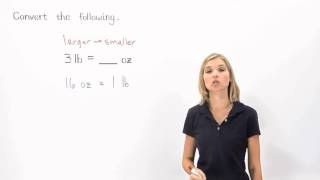By MathHelp.com

This lesson covers complex numbers. Students learn that a complex number is the sum or difference of a real number and an imaginary number and can be written in a + bi form. For example, 1 + 2i and -- 5 - i root 7 are complex numbers. Students then learn to add, subtract, multiply, and divide complex numbers that do not contain radicals, such as (5 + 3i) / (6 - 2i). To divide (5 + 3i) / (6 - 2i), the first step is to multiply both the numerator and denominator of the fraction by the conjugate of the denominator, which is (6 + 2i), then FOIL in both the numerator and denominator, and combine like terms.##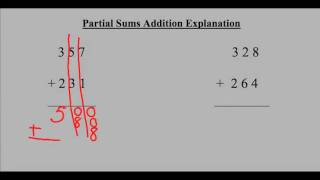By Mathademics# Addition of Fractions | Maths for Kids | Grade 5 | Periwinkle

##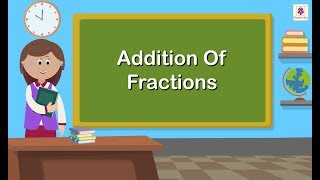By Periwinkle# One-step addition and subtraction equations with fractions and decimals

##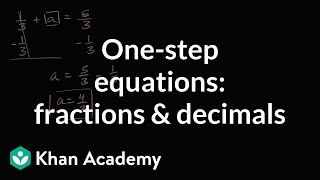By Khan Academy

Learn how to solve one-step addition and subtraction equations that have fractions and decimals in them.# One-step addition and subtraction equations with fractions and decimals

##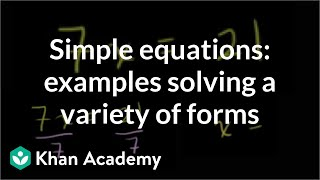By Khan Academy

Some quick examples to practice solving a variety of one step equations. All 4 operations (add, subtract, multiple, divide) are paired with variables.# One-step addition and subtraction equations with fractions and decimals

##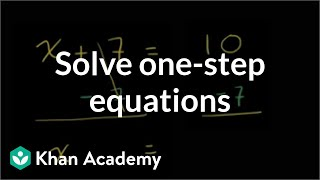By Khan Academy

Learn how to solve one-step addition and subtraction equations by adding or subtracting the same thing from both sides of the equation.# One-step addition and subtraction equations with fractions and decimals

##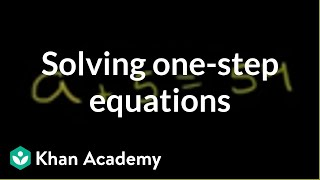By Khan Academy

Learn how to solve this equation: a + 5 = 54ï¿½ï¿½ï¿½ï¿½ï¿½ï¿½ï¿½ï¿½ï¿½ï¿½ï¿½ï¿½# Comparing decimals: difference in largest place value | Decimals | Pre-Algebra | Khan Academy

##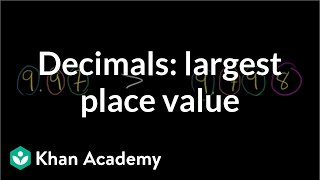By Khan Academy

Confused trying to determine which decimal is larger? Let's compare two decimals in this example. Remember that you're looking for the difference is the largest place value to find the larger decimal.##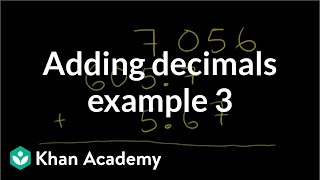By Khan Academy

We're adding 3 decimal numbers together in this example. Give it a try!# Adding and subtracting decimals word problems

##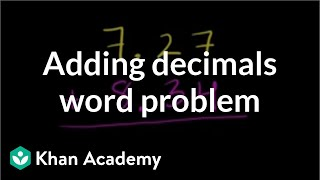By Khan Academy

Here's a simple word problem asking us to put our adding decimals skill to work. We sound like a broken record, but be sure and ALIGN DECIMALS.# Adding and subtracting decimals word problems

##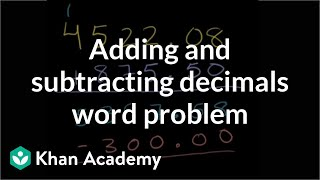By Khan Academy

Let's get fancy and practice adding and subtracting decimals in the same word problem.# Comparing decimals: place value difference | Decimals | Pre-Algebra | Khan Academy

##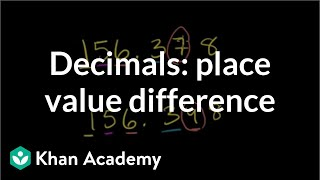By Khan Academy

It's not uncommon to be asked to compare two decimals and judge which one is larger. How do you do that? This video will gave you the confidence to answer that question.# Estimating Sums and Differences | MathHelp.com

##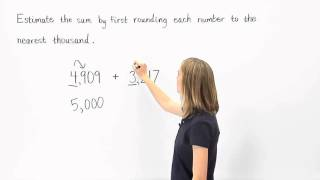By MathHelp.com# How To Derive The Formula For The Sum of an Arithmetic Series

##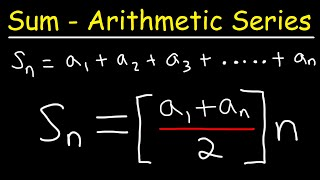By The Organic Chemistry Tutor##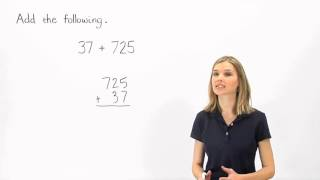By MathHelp.com# Associative Property of Addition | MathHelp.com

##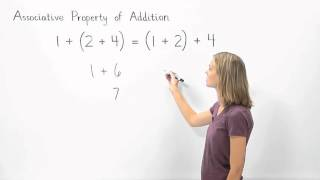By MathHelp.com# Multiplying Decimals - On a Place Value Chart (5.NBT.B.7)

##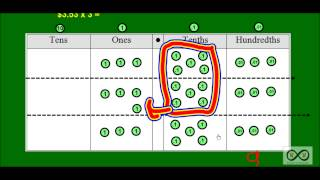By Worksheets and Walkthroughs

This video walkthrough lesson with corresponding worksheets investigates what happens on a place value chart as we multiply a decimal by a single digit number in a story problem format. Place value disks are used to represent values on a place value chart.# The Arithmetic Series - Part 1 - 

##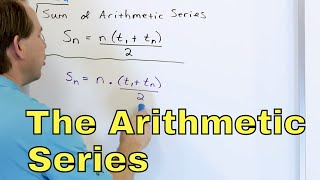By Math and Science# Angles in Triangles

##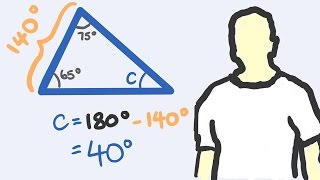By tecmath